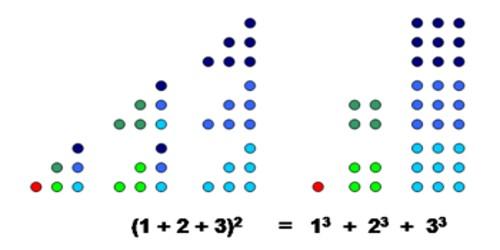Evaluate Squares and Cubes

Evaluate Squares

An exponent is a number that tells how many times the base number is used as a factor. For example, 32 indicates that the base number 3 is used as a factor 2 times. To determine the value of 32, multiply 3*3 which would give the result 9.

Squares indicate that the exponent has a value of two. The term square comes from the geometrical shape that has the same width and length. To find the area of a square you would multiply the width times the length.

Exponents are written as a superscript number (e.g. 32) or preceded by the caret (^) symbol (e.g. 3^2).

Zero squared is zero (e.g. 02 = 0)

One squared is one (e.g. 12 = 1)The Cube of a Number

An exponent is a number that tells how many times the base number is used as a factor. For example, 43 indicates that the base number 4 is used as a factor 3 times. To determine the value of 43, multiply 4*4*4 which would give the result 64.

Cubes indicate that the exponent has a value of three. The term cube comes from the geometrical shape that has the same width and length and height. To find the volume of a cube you would multiply the width times the length times the height.

Exponents are written as a superscript number (e.g. 43) or preceded by the caret (^) symbol (e.g. 4^3).